Question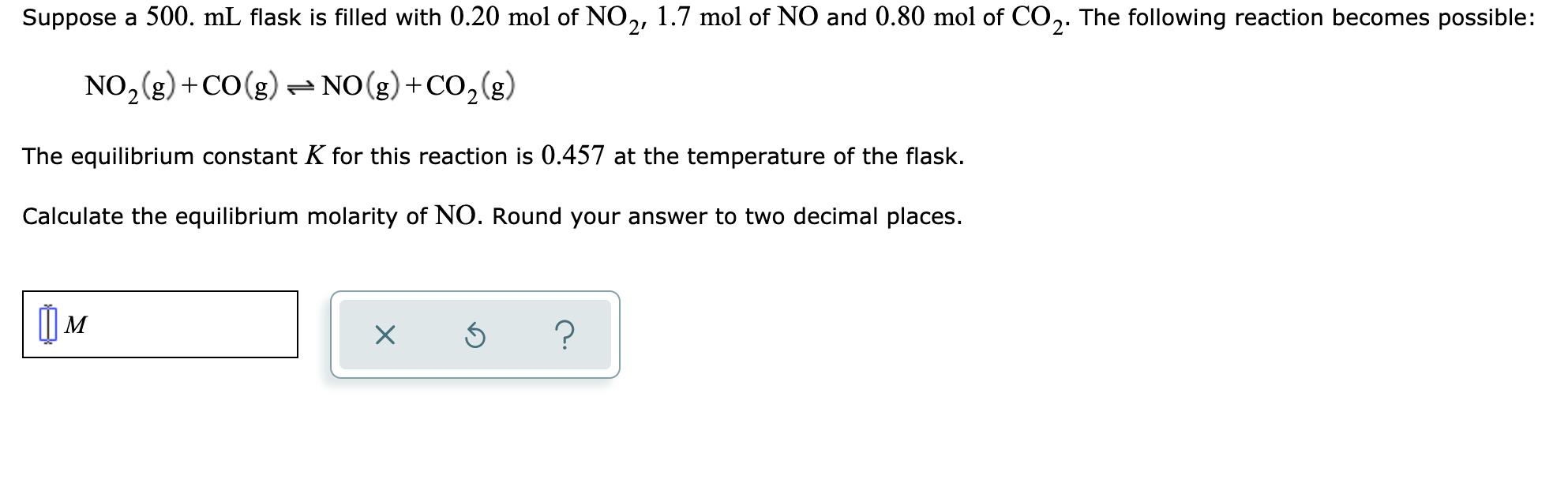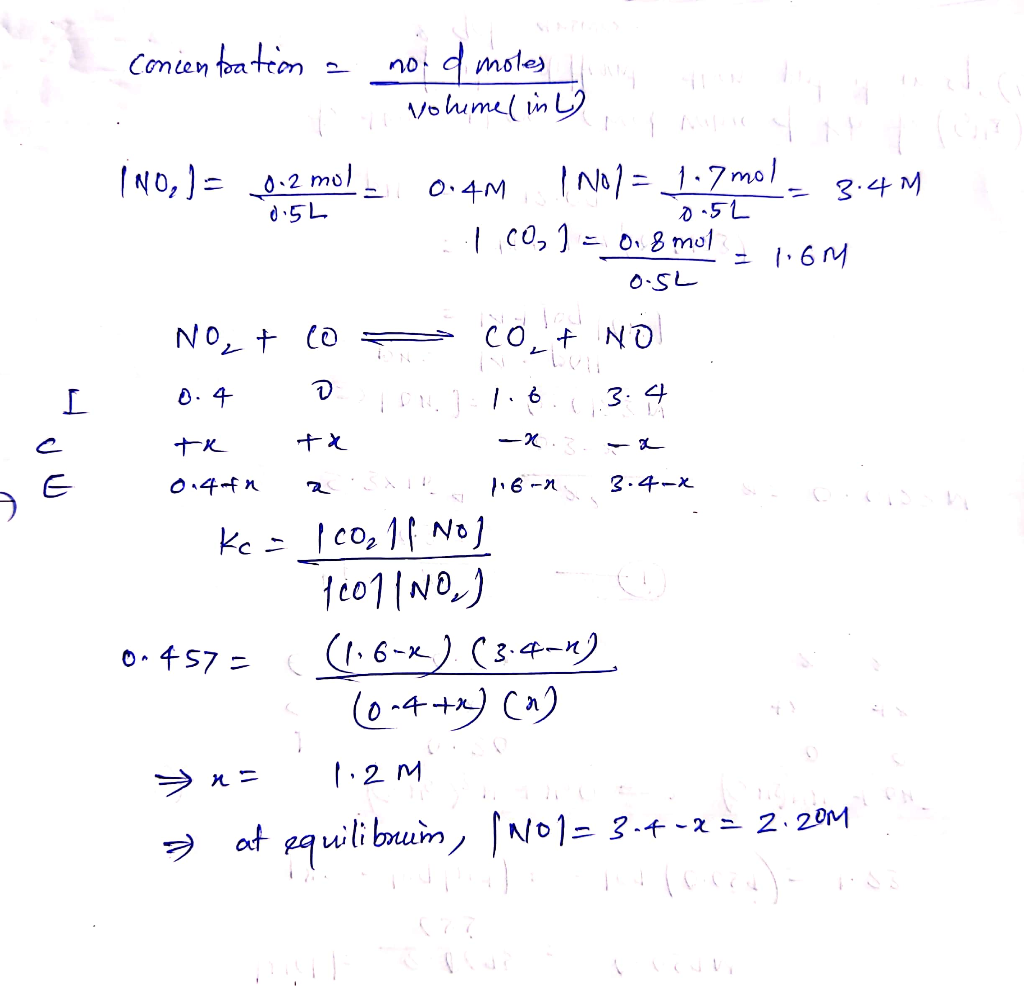#### Earn Coins

Coins can be redeemed for fabulous gifts.

Similar Homework Help Questions
• ### Suppose a 500. mL flask is filled with 0.70 mol of NO2, 2.0 mol of NO...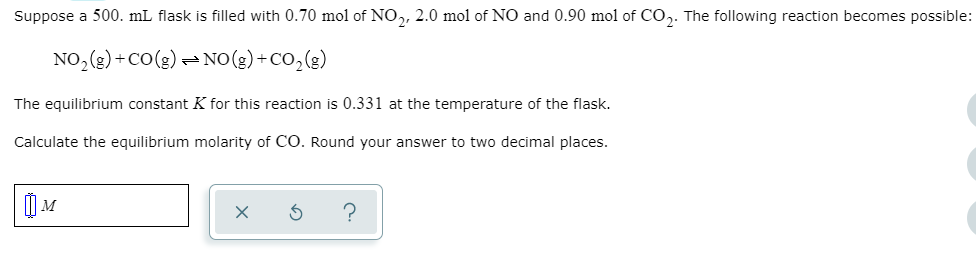Suppose a 500. mL flask is filled with 0.70 mol of NO2, 2.0 mol of NO and 0.90 mol of CO2. The following reaction becomes possible: NO2(e)+Co(g)NO(g)+Co,(g) The equilibrium constant K for this reaction is 0.331 at the temperature of the flask. Calculate the equilibrium molarity of CO. Round your answer to two decimal places.

• ### Suppose a 500 ml flask is filled with 0.50 mol of CO, 1.7 mol of H,0...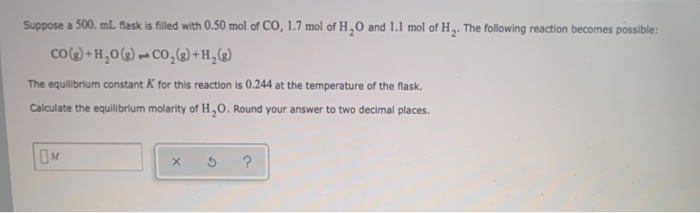Suppose a 500 ml flask is filled with 0.50 mol of CO, 1.7 mol of H,0 and 1.1 mol of H. The following reaction becomes possible: CO(g) +H,0(8) -CO2() +H,() The equilibrium constant K for this reaction is 0.244 at the temperature of the flask. Calculate the equilibrium molarity of 1,0. Round your answer to two decimal places.

• ### Suppose a 500. mL flask is filled with 0.40 mol of CO, 0.60 mol of NO...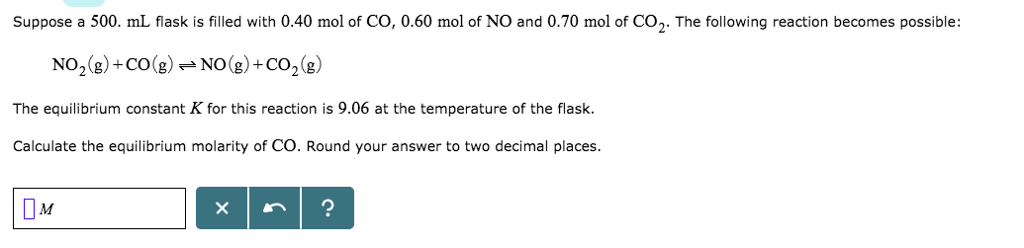Suppose a 500. mL flask is filled with 0.40 mol of CO, 0.60 mol of NO and 0.70 mol of CO2. The following reaction becomes possible: NO2(g) +CONO( CO2() The equilibrium constant K for this reaction is 9.06 at the temperature of the flask. Calculate the equilibrium molarity of CO. Round your answer to two decimal places. Џи

• ### Suppose a 500. mL flask is filled with 1.5 mol of CO, 1.2 mol of NO...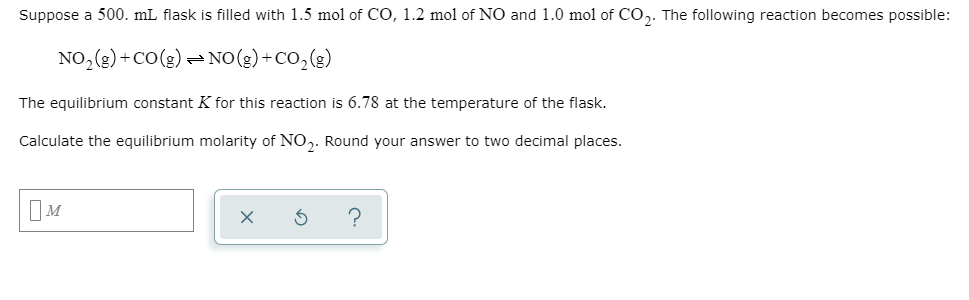Suppose a 500. mL flask is filled with 1.5 mol of CO, 1.2 mol of NO and 1.0 mol of CO,. The following reaction becomes possible: NO2(g) +CO(g) = NO(g) + CO2(g) The equilibrium constant K for this reaction is 6.78 at the temperature of the flask. Calculate the equilibrium molarity of NO2. Round your answer to two decimal places. Пм x 6 ?

• ### Suppose a 500. ml flask is filled with 0.90 mol of NO,, 0.10 mol of CO...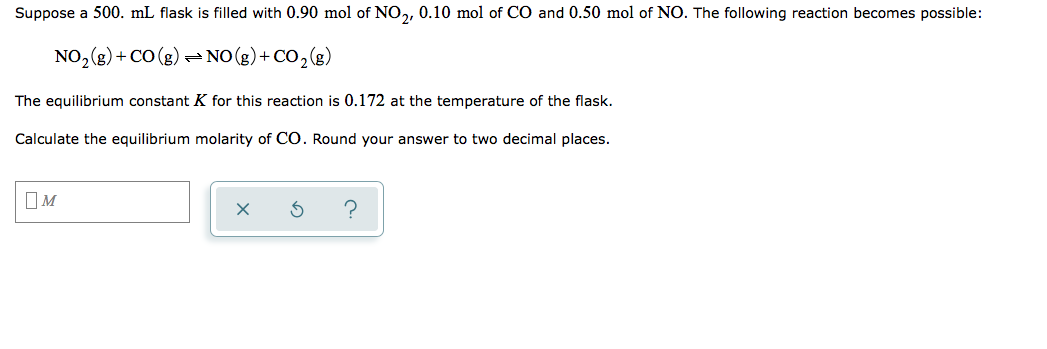Suppose a 500. ml flask is filled with 0.90 mol of NO,, 0.10 mol of CO and 0.50 mol of NO. The following reaction becomes possible: NO2(g) +CO(g) = NO(g) + CO2(g) The equilibrium constant K for this reaction is 0.172 at the temperature of the flask. Calculate the equilibrium molarity of CO. Round your answer to two decimal places. IM xs ?

• ### Suppose a 500. ml flask is filled with 0.90 mol of NO, and 0.80 mol of...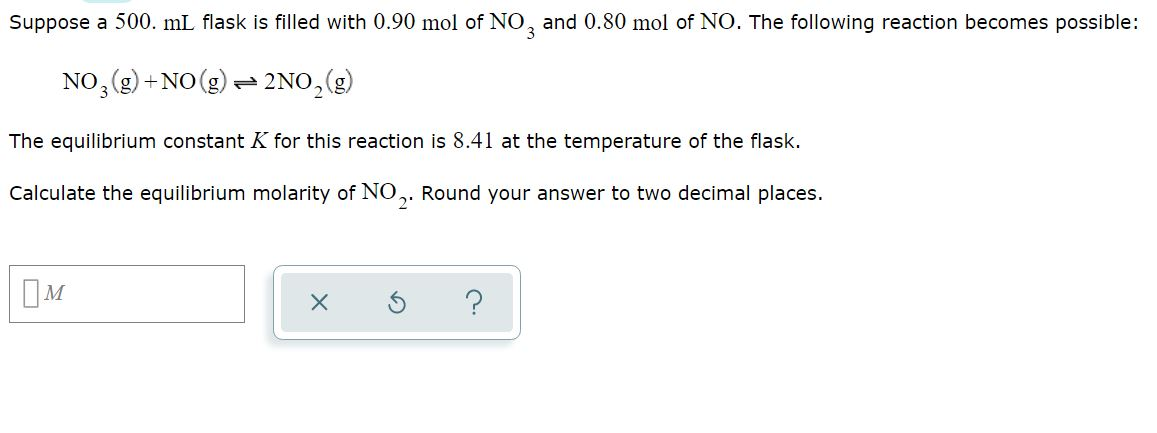Suppose a 500. ml flask is filled with 0.90 mol of NO, and 0.80 mol of NO. The following reaction becomes possible: NO2(g) + NO(g) + 2NO, (g) The equilibrium constant K for this reaction is 8.41 at the temperature of the flask. Calculate the equilibrium molarity of NO. Round your answer to two decimal places. IM x 3 ?

• ### Suppose a 500.mL flask is filled with 1.1mol of NO2 1.6mol of CO and 0.90mol of...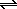Suppose a 500.mL flask is filled with 1.1mol of NO2 1.6mol of CO and 0.90mol of NO. The following reaction becomes possible: NO2(g) + CO(g) NO(g) +CO2(g) The equilibrium constant K for this reaction is 0.211 at the temperature of the flask. Calculate the equilibrium molarity of NO. Round your answer to two decimal places.

• ### Suppose a 500 ml flask is filled with 1.7 mol of CO, 0.40 mol of H.O...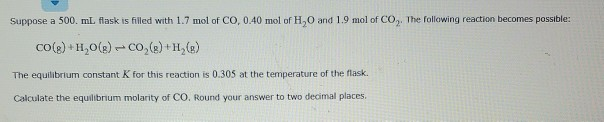Suppose a 500 ml flask is filled with 1.7 mol of CO, 0.40 mol of H.O and 1.9 mol of CO,The following reaction becomes possible: CO(e)+H,0(B) - CO (8)+H.) The equilibrium constant K for this reaction is 0.305 at the temperature of the flask. Calculate the equilibrium molarity of CO. Round your answer to two decimal places

• ### Suppose a 500. mL flask is filled with 0.50 mol of H, and 1.7 mol of...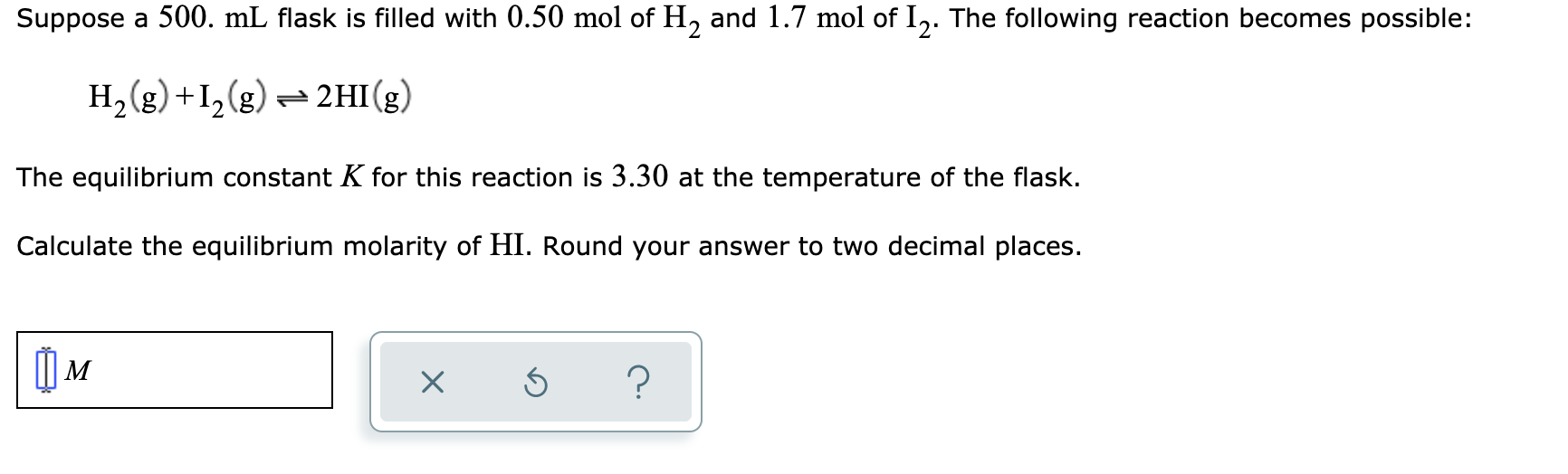Suppose a 500. mL flask is filled with 0.50 mol of H, and 1.7 mol of 12. The following reaction becomes possible: H2(g) +12(g) = 2HI(g) The equilibrium constant K for this reaction is 3.30 at the temperature of the flask. Calculate the equilibrium molarity of HI. Round your answer to two decimal places. xs ?

• ### Suppose a 500 ml flask is filled with 1.6 mol of H, and 1.7 mol of...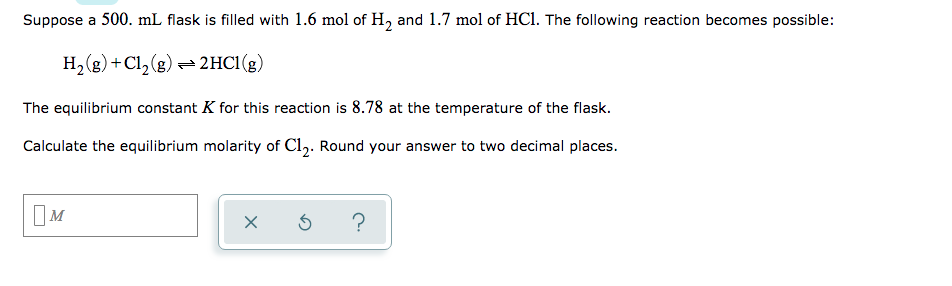Suppose a 500 ml flask is filled with 1.6 mol of H, and 1.7 mol of HCl. The following reaction becomes possible: H2(g) +C12(g) + 2HCI(g) The equilibrium constant K for this reaction is 8.78 at the temperature of the flask. Calculate the equilibrium molarity of Cl,. Round your answer to two decimal places. OM x 6 ?